STAT 330: 95-3

Midterm Solutions

1. Q1

1. Let p be the proportion of all adult Quebec voters who would say they would vote no if asked. You are to decide between p > 0.5 and p < 0.5. To collect evidence in favour of the referendum failing we must test the opposite hypothesis and assess the strength of evidence against that null. Namely, we define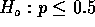. The value of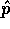is 465/900 and our test statistic is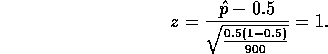The P-value is the area in the right hand tail since we would reject for large z values. We find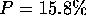and conclude that there is only weak evidence that the referendum will not pass.

2. Now letbe the p of part (a) and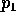be the same proportion but one week earlier in time. We are to test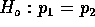against the alternative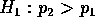. If the null hypothesis is true we estimate p, the common value ofandby pooling the two surveys to get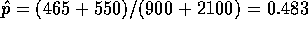. Our test statistic isand the one-tailed P-value is P= 0.0008 so that we conclude that the proportion supporting NO has definitely increased.

3. Here I was looking for comments on the fact that most surveys have significant non-response rates, and response bias and the fact that most are not simple random samples but cluster samples. I did not want to see `the population is normal' because there is NO continuous variable around which could be normal.

2. Q2

1. Let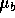be the true weight. Then assuming that the measurements have mean, that measurement errors have a normal distribution and that replicate measurements may be treated as independent we can use a t confidence interval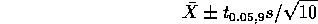which is, in units of micrograms above 10 grams, 496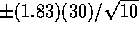.

2. Now let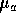be the weight after dropping. We test the hypothesis that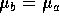by using a 2 sample t-test. The pooled estimate of the variance is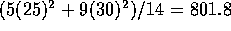so the test statistic is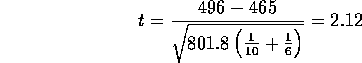which leads to a two sided P value of 0.052. (From the tables you can tell that P is very close to 0.05 and a bit bigger than 0.05.) Thus we conclude that there is rather marginal evidence of a change in weight.

3. This is a sample size calculation. You are told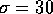,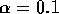and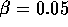. The alternative is two sided and the formula, from the text, for the sample size is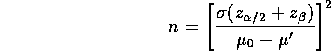The questions gives the denominator the value 5 so we get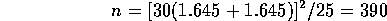roughly.

3. Q3

1.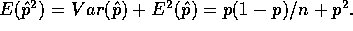2.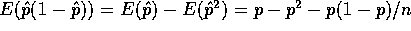so that the bias is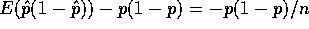.
3. The basic principle is that the mle of a function of a parameter is that function applied to the parameter so that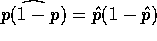where of course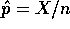.

Richard Lockhart
Wed Feb 11 09:09:27 PST 1998